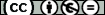## A von Staudt-type result for $\displaystyle{\sum_{z\in\mathbb{Z}_n[i]} z^k }$

Ayuso, Pedro ; Grau, José María ; Oller Marcén, Antonio M.
Resumen: n this paper we study the sum of powers of the Gaussian integers Gk(n):=¿a,b¿[1,n](a+bi)k. We give an explicit formula for Gk(n)(modn) in terms of the prime numbers p=3(mod4) with p||n and p-1|k, similar to the well known one due to von Staudt for ¿ni=1ik(modn). We apply this result to study the set of integers n which divide Gn(n) and compute its asymptotic density with six exact digits: 0.971000….
Idioma: Inglés
DOI: 10.1007/s00605-015-0736-5
Año: 2015
Publicado en: MONATSHEFTE FUR MATHEMATIK 178, 3 (2015), 345-359
ISSN: 0026-9255

Factor impacto JCR: 0.664 (2015)
Categ. JCR: MATHEMATICS rank: 131 / 311 = 0.421 (2015) - Q2 - T2
Factor impacto SCIMAGO:

Tipo y forma: Article (PostPrint)You must give appropriate credit, provide a link to the license, and indicate if changes were made. You may do so in any reasonable manner, but not in any way that suggests the licensor endorses you or your use. You may not use the material for commercial purposes. If you remix, transform, or build upon the material, you may not distribute the modified material.

Este artículo se encuentra en las siguientes colecciones:
Articles